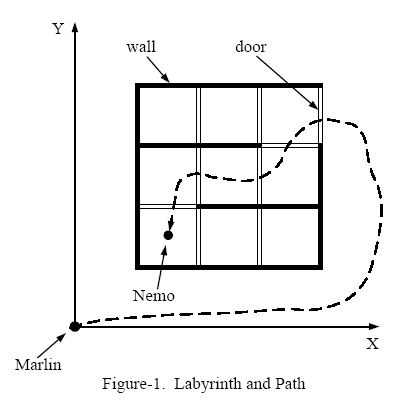# POJ 2049-Finding Nemo(三维bfs解决类迷宫问题)

Finding Nemo
 Time Limit: 2000MS Memory Limit: 30000K Total Submissions: 7902 Accepted: 1827

Description

Nemo is a naughty boy. One day he went into the deep sea all by himself. Unfortunately, he became lost and couldn't find his way home. Therefore, he sent a signal to his father, Marlin, to ask for help.
After checking the map, Marlin found that the sea is like a labyrinth with walls and doors. All the walls are parallel to the X-axis or to the Y-axis. The thickness of the walls are assumed to be zero.
All the doors are opened on the walls and have a length of 1. Marlin cannot go through a wall unless there is a door on the wall. Because going through a door is dangerous (there may be some virulent medusas near the doors), Marlin wants to go through as few doors as he could to find Nemo.
Figure-1 shows an example of the labyrinth and the path Marlin went through to find Nemo.We assume Marlin's initial position is at (0, 0). Given the position of Nemo and the configuration of walls and doors, please write a program to calculate the minimum number of doors Marlin has to go through in order to reach Nemo.

Input

The input consists of several test cases. Each test case is started by two non-negative integers M and N. M represents the number of walls in the labyrinth and N represents the number of doors.
Then follow M lines, each containing four integers that describe a wall in the following format:
x y d t
(x, y) indicates the lower-left point of the wall, d is the direction of the wall -- 0 means it's parallel to the X-axis and 1 means that it's parallel to the Y-axis, and t gives the length of the wall.
The coordinates of two ends of any wall will be in the range of [1,199].
Then there are N lines that give the description of the doors:
x y d
x, y, d have the same meaning as the walls. As the doors have fixed length of 1, t is omitted.
The last line of each case contains two positive float numbers:
f1 f2
(f1, f2) gives the position of Nemo. And it will not lie within any wall or door.
A test case of M = -1 and N = -1 indicates the end of input, and should not be processed.

Output

For each test case, in a separate line, please output the minimum number of doors Marlin has to go through in order to rescue his son. If he can't reach Nemo, output -1.

Sample Input

8 9
1 1 1 3
2 1 1 3
3 1 1 3
4 1 1 3
1 1 0 3
1 2 0 3
1 3 0 3
1 4 0 3
2 1 1
2 2 1
2 3 1
3 1 1
3 2 1
3 3 1
1 2 0
3 3 0
4 3 1
1.5 1.5
4 0
1 1 0 1
1 1 1 1
2 1 1 1
1 2 0 1
1.5 1.7
-1 -1

Sample Output

5
-1

#include <stdio.h>
#include <string.h>
#include <stdlib.h>
#include <iostream>
#include <algorithm>
#include <queue>
using namespace std;
const int inf=0x3f3f3f3f;
struct node {
int x, y;
int ans;
} f1,f2;
int map;
int vis;
int jx[]= {0,0,1,-1};
int jy[]= {1,-1,0,0};
int min1;
void bfs(int s, int e)
{
int i, j;
queue<node>q;
f1.x=s;
f1.y=e;
f1.ans=0;
vis[s][e]=1;
q.push(f1);
min1=inf;
while(!q.empty()) {
f1=q.front();
q.pop();
if(f1.x<=0||f1.x>=199||f1.y<=0||f1.y>=199) {//边界处理，但是不能跳出，只能跳过，因为存在多个门的情况
min1=min(min1,f1.ans); //走出去之后，保存最小通过门数
continue ;
}
for(i=0; i<4; i++) {
f2.x=f1.x+jx[i];
f2.y=f1.y+jy[i];
if(i==0) {//向上走
if(!vis[f2.x][f2.y]&&map[f1.x][f1.y]!=2) {
if(map[f1.x][f1.y]==1)
f2.ans=f1.ans+1;
else
f2.ans=f1.ans;
vis[f2.x][f2.y]=1;
q.push(f2);
}
} else if(i==1) {//向下走
if(!vis[f2.x][f2.y]&&map[f2.x][f2.y]!=2) {
if(map[f2.x][f2.y]==1)
f2.ans=f1.ans+1;
else
f2.ans=f1.ans;
vis[f2.x][f2.y]=1;
q.push(f2);
}
} else if(i==2) {//向右走
if(!vis[f2.x][f2.y]&&map[f1.x][f1.y]!=2) {
if(map[f1.x][f1.y]==1)
f2.ans=f1.ans+1;
else
f2.ans=f1.ans;
vis[f2.x][f2.y]=1;
q.push(f2);
}
} else if(i==3) {//向左走
if(!vis[f2.x][f2.y]&&map[f2.x][f2.y]!=2) {
if(map[f2.x][f2.y]==1)
f2.ans=f1.ans+1;
else
f2.ans=f1.ans;
vis[f2.x][f2.y]=1;
q.push(f2);
}
}
}
}
}
int main()
{
int n, m, i, j;
int x,y,d,t;
double a1,a2;
int int_x,int_y;
while(scanf("%d %d",&m,&n)!=EOF) {
if(n==-1&&m==-1) break;
memset(map,0,sizeof(map));
memset(vis,0,sizeof(vis));
for(i=0; i<m; i++) {
scanf("%d %d %d %d",&x, &y, &d, &t);
if(d) {
for(j=0; j<t; j++) {
map[x-1][y+j]=2;
}
} else {
for(j=0; j<t; j++) {
map[x+j][y-1]=2;
}
}
}
for(i=0; i<n; i++) {
scanf("%d %d %d",&x,&y,&d);
if(d) {
map[x-1][y]=1;
} else {
map[x][y-1]=1;
}
}
scanf("%lf %lf",&a1,&a2);
int_x=a1;
int_y=a2;
if(int_x<=0||int_x>=199||int_y<=0||int_y>=199) {//后台可能出现 0,0 200+,200+，虽然不符合题目描述
printf("0\n");
continue ;
}
bfs(int_x,int_y);
if(min1==inf)
printf("-1\n");
else
printf("%d\n",min1);
}
return 0;
}


©️2019 CSDN 皮肤主题: 酷酷鲨 设计师: CSDN官方博客